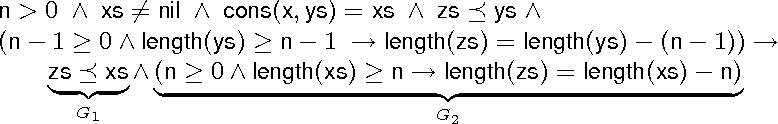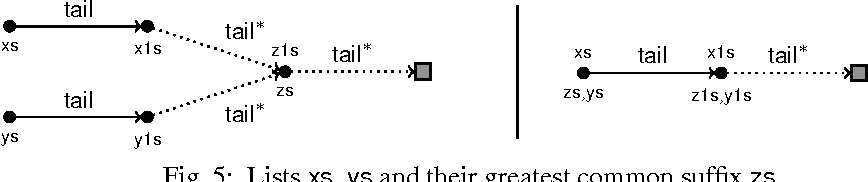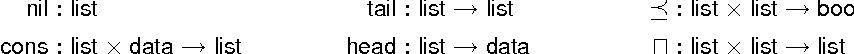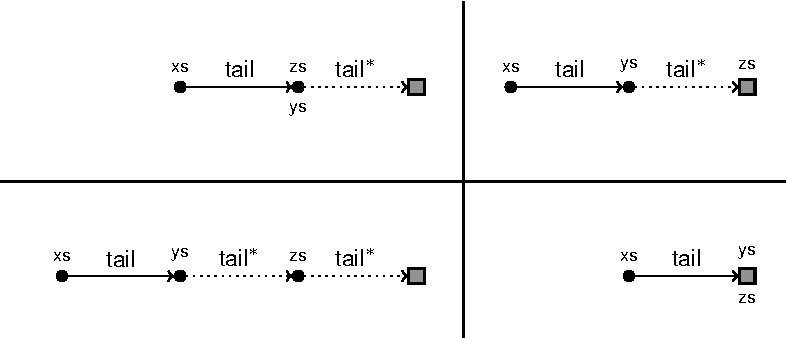You are currently offline. Some features of the site may not work correctly.

# List of NP-complete problems

Known as: List of np complete problems, Solvability of equations
This is a list of some of the more commonly known problems that are NP-complete when expressed as decision problems. As there are hundreds of such… Expand
Wikipedia

## Papers overview

Semantic Scholar uses AI to extract papers important to this topic.
2014
2014
The monodromy group of an algebraic function is isomorphic to the Galois group of the associated extension of the field of… Expand
Is this relevant?
2013
2013
• 2013
• Corpus ID: 119143506
Fix an odd prime \$p\$, and let \$F\$ be a field containing a primitive \$p\$th root of unity. It is known that a \$p\$-rigid field \$F… Expand
Is this relevant?
2012
2012
• VSTTE
• 2012
• Corpus ID: 2199192
Motivated by the problem of deciding verification conditions for the verification of functional programs, we present new decision… Expand
••••Is this relevant?
2007
2007
Second order parabolic equations in Sobolev spaces with mixed norms are studied. The leading coefficients (except a11) are… Expand
Is this relevant?
2006
2006
• Int. J. Algebra Comput.
• 2006
• Corpus ID: 1975140
We show that the existential theory of free partially commutative monoids with involution is decidable. As a consequence the… Expand
Is this relevant?
2001
2001
• 2001
• Corpus ID: 115383828
AbstractWe investigate the solvability of equations Q(f,f)+∈2Δf=0 in term of nonnegative integrable densities f∈L1+(R3). Here… Expand
Is this relevant?
1998
1998
• C. Gutiérrez
• Proceedings 39th Annual Symposium on Foundations…
• 1998
• Corpus ID: 14273125
In this paper we study solvability of equations over free semigroups, known as word equations, particularly G.S. Makanin's… Expand
Is this relevant?
1987
1987
• Arch. Math. Log.
• 1987
• Corpus ID: 31157052
We will show (Theorem I) that every existentially definable relation over concatenation is diophantine. That is, it can be… Expand
Is this relevant?
Highly Cited
1977
Highly Cited
1977
In this paper we construct an algorithm recognizing the solvability of arbitrary equations in a free semigroup.Bibliography: 4… Expand
Is this relevant?
Highly Cited
1975
Highly Cited
1975
Introduction 1. .4-proper mappings and fixed point theorems for Py-compact mappings 1.1 Basic facts about ^-proper mappings and… Expand
Is this relevant?